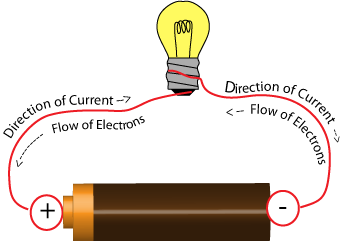# What is electric current?

When electric charges move from one point to another, we call it electric current.

In a conductor, an electric current is the rate of flow of electrons.

The SI Unit of electric current is the Ampere.Ohm's law gives the relationship between current, voltage, and resistance.

Mathematically this relationship is given as,

$V = I \times R$, where

V = voltage across resistor

I = current

R = resistance

Updated on: 10-Oct-2022

58 Views